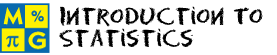# Introduction to StatisticsThis page lists the Learning Objectives for all lessons in Unit 8.

#### Range

The student will be able to:

• Define data and range.
• Examine a real-world problem in which the range of a set of whole numbers is found.
• Examine a real-world problem in which the range of a set of decimals is found.
• Examine a real-world problem in which the range of a set of integers is found.
• Compute the range of a set of numbers.
• Describe the procedure for computing the range of a set of numbers.
• Apply range procedures to complete five interactive exercises.

#### Mean

The student will be able to:

• Define arithmetic mean.
• Examine real-world problems in which the mean of a set of whole numbers is computed.
• Examine real-world problems in which the mean of a set of decimals is computed.
• Describe the procedure for finding the mean of a set of numbers.
• Compute the mean of a set of numbers.
• Apply arithmetic mean procedures to complete five interactive exercises.

#### Non-Routine Mean

The student will be able to:

• Examine non-routine problems that involve an arithmetic mean.
• Determine the sum of n numbers given the mean and the value of n.
• Determine n given the sum of n numbers and the mean.
• Determine the missing number in a set of data given the mean and the other numbers in that set.
• Examine non-routine problems involving an arithmetic mean.
• Apply non-routine mean procedures to complete five interactive exercises.

#### Median

The student will be able to:

• Define median.
• Examine real-world problems in which the median of a set of whole numbers is computed.
• Examine real-world problems in which the median of a set of decimals is computed.
• Differentiate between finding the median of an odd number of items and an even number of items.
• Describe the procedure for finding the median of a set of data.
• Compute the median for an odd number of items and for an even number of items.
• Apply median procedures to complete five interactive exercises.

#### Mode

The student will be able to:

• Define mode and bimodal.
• Examine real-world problems in which the mode of a set of whole numbers, decimals or integers is computed.
• Examine problems for which there is no mode.
• Examine problems for which the data is bimodal.
• Examine problems for which the mode is zero.
• Differentiate between sets of data with no mode, a mode of zero and two modes.
• Determine the mode of a set of data.
• Apply mode procedures to complete five interactive exercises.

#### Practice Exercises

The student will be able to:

• Examine ten interactive exercises for all topics in this unit.
• Determine which concepts and procedures are needed to complete each practice exercise.
• Compute answers by applying appropriate concepts and procedures.
• Self-assess knowledge and skills acquired from this unit.

#### Challenge Exercises

The student will be able to:

• Evaluate ten interactive exercises with word problems for all topics in this unit.
• Analyze each problem to identify the given information.
• Formulate a strategy for solving each problem.
• Apply strategies to solve routine and non-routine problems.
• Synthesize all information presented in this unit.
• Identify connections between basic statistics and the real world.
• Develop problem-solving skills.

#### Solutions

The student will be able to:

• Examine the solution for each exercise presented in this unit.
• Identify which solutions need to be reviewed.
• Compare solutions to completed exercises.
• Identify and evaluate incorrect answers.
• Amend and label original answers.
• Identify areas of strength and weakness.
• Decide which concepts and procedures need to be reviewed from this unit.

### Order your CD today for Home or SchoolOR